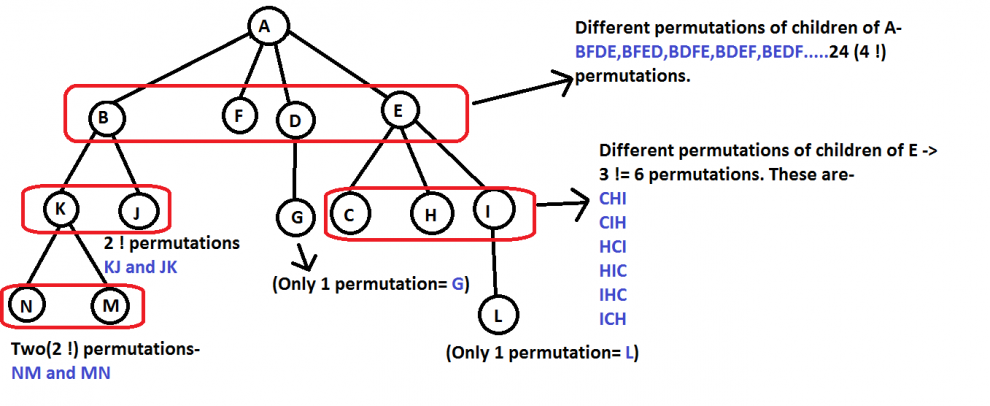# Python Program – Inorder Tree Traversal without Recursioninorder tree travesal without recursion - Using Stack is the obvious way to traverse tree without recursion.Below is an algorithm for traversing binary tree

Using Stack is the obvious way to traverse tree without recursion. Below is an algorithm for traversing binary tree using stack. See this for step wise step execution of the algorithm.

```1) Create an empty stack S.
2) Initialize current node as root
3) Push the current node to S and set current = current->left until current is NULL
4) If current is NULL and stack is not empty then
a) Pop the top item from stack.
b) Print the popped item, set current = popped_item->right
c) Go to step 3.
5) If current is NULL and stack is empty then we are done.
```

Let us consider the below tree for example

```            1
/   \
2      3
/  \
4     5

Step 1 Creates an empty stack: S = NULL

Step 2 sets current as address of root: current -> 1

Step 3 Pushes the current node and set current = current->left until current is NULL
current -> 1
push 1: Stack S -> 1
current -> 2
push 2: Stack S -> 2, 1
current -> 4
push 4: Stack S -> 4, 2, 1
current = NULL

Step 4 pops from S
a) Pop 4: Stack S -> 2, 1
b) print "4"
c) current = NULL /*right of 4 */ and go to step 3
Since current is NULL step 3 doesn't do anything.

Step 4 pops again.
a) Pop 2: Stack S -> 1
b) print "2"
c) current -> 5/*right of 2 */ and go to step 3

Step 3 pushes 5 to stack and makes current NULL
Stack S -> 5, 1
current = NULL

Step 4 pops from S
a) Pop 5: Stack S -> 1
b) print "5"
c) current = NULL /*right of 5 */ and go to step 3
Since current is NULL step 3 doesn't do anything

Step 4 pops again.
a) Pop 1: Stack S -> NULL
b) print "1"
c) current -> 3 /*right of 5 */

Step 3 pushes 3 to stack and makes current NULL
Stack S -> 3
current = NULL

Step 4 pops from S
a) Pop 3: Stack S -> NULL
b) print "3"
c) current = NULL /*right of 3 */```
``````# Python program to do inorder traversal without recursion

# A binary tree node
class Node:

# Constructor to create a new node
def __init__(self, data):
self.data = data
self.left = None
self.right = None

# Iterative function for inorder tree traversal
def inOrder(root):

# Set current to root of binary tree
current = root
s = [] # initialze stack
done = 0

while(not done):

# Reach the left most Node of the current Node
if current is not None:

# Place pointer to a tree node on the stack
# before traversing the node's left subtree
s.append(current)

current = current.left

# BackTrack from the empty subtree and visit the Node
# at the top of the stack; however, if the stack is
# empty you are done
else:
if(len(s) >0 ):
current = s.pop()
print current.data,

# We have visited the node and its left
# subtree. Now, it's right subtree's turn
current = current.right

else:
done = 1

# Driver program to test above function

""" Constructed binary tree is
1
/   \
2     3
/  \
4    5   """

root = Node(1)
root.left = Node(2)
root.right = Node(3)
root.left.left = Node(4)
root.left.right = Node(5)

inOrder(root)

# This code is contributed by Nikhil Kumar Singh(nickzuck_007)``````

READ  C program -Level Order Tree Traversal#### Venkatesan Prabu

Wikitechy Founder, Author, International Speaker, and Job Consultant. My role as the CEO of Wikitechy, I help businesses build their next generation digital platforms and help with their product innovation and growth strategy. I'm a frequent speaker at tech conferences and events.

X